Problems & Puzzles: Puzzles

Puzzle 290.  Primes on Platonic solid faces

On my request Frank Rubin proposed one puzzle for my pages:

It is possible to write a prime on each face of a cube so that the 3 faces surrounding each vertex sum to a prime.  For example, if you write 3 on a pair of opposite faces, and 2 on the other 4 faces, then the 3 primes around each vertex sum to 7.  Find the set of 6 distinct primes with this property such that their sum is minimal.

The particular question posed by Rubin is not really hard to find even without a computer program. A little harder problem is to find a solution of minimal sum using six consecutive primes.

...

What if we ask both questions for those platonic solids who have an odd number of faces contiguous to each vertex - tetrahedron, cube (or hexahedron), dodecahedron and icosahedron?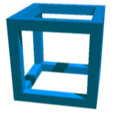(shown here thanks to the Rüdiger Appel's page)

In order to organize the search of the answers to this puzzle we need to use the planar projection of the platonic solids as follows:

 Tetrahedron Hexahedron Dodecahedron Icosahedron Faces contiguous to each vertex 3 3 3 5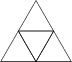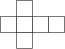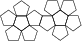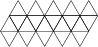Please download this Excel worksheet in order to see the variable names that I propose for each face and each vertex, for each platonic solid planar projection. Please use this proposal in order to compare easily the distinct solutions gotten by different puzzlers.Example. For a tetrahedron (faces: a, b, c, d; vertexes: A, B, C, D)

1) Using distinct primes for the faces, the minimal sum is gotten with the following values:

a = 5, b = 7, c = 17, d = 19, S = a+b+c+d = 48
A=a+b+c = 29, B=a+b+d=31, C=a+c+d=41, D=b+c+d=43

2) Using consecutive primes for the faces, the minimal sum is gotten with the following values:

a = 19, b = 23, c = 29, d = 31, S = a+b+c+d = 102
A=a+b+c = 71, B=a+b+d=73, C=a+c+d=79, D=b+c+d=83

Questions:

1. Find a solution for the remaining three platonic solids (cube, dodecahedron, icosahedron) such that the sum of all the primes in the faces, S, is minimal: 1) using distinct primes 2) using consecutive primes.

2. Explain why no solution exist for a tetrahedron using distinct primes, one of each being the prime '3'.Contributions came from Torbjörn Alm and Ray Opao

Torbjörn Alm solved completely the question 1.

1.1) Distinct primes

 Cube Dodecahedron Icosahedron Sum = 72 Faces: a:3 b:5 c:13 d:17 e:11 f:23 Vertices: A = e+a+b = 19 B = e+b+c = 29 C = e+c+d = 41 D = e+d+a = 31 E = f+a+b = 31 F = f+b+c = 41 G = f+c+d = 53 H = f+d+a = 43 Sum = 272 Faces: a:3 b:5 c:31 d:19 e:17 f:41 g:29 h:37 i:11 j:43 k:23 l:13 Vertices: A = k+e+a = 43 B = k+a+b = 31 C = k+b+c = 59 D = k+c+d = 73 E = k+d+e = 59 F = l+f+g = 83 G = l+g+h = 79 H = l+h+i = 61 I = l+i+j = 67 J = l+j+f = 97 K = a+f+g = 73 L = b+g+h = 71 M = c+h+i = 79 N = d+i+j = 73 O = e+j+f = 101 P = f+e+a = 61 Q = g+a+b = 37 R = h+b+c = 73 S = i+c+d = 61 T = j+d+e = 79 Same as with consecutive primes

1.2) Consecutive primes

 Cube Dodecahedron Icosahedron Sum = 90 Faces: a:7 b:11 c:23 d:17 e:13 f:19 Vertices: A = e+a+b = 31 B = e+b+c = 47 C = e+c+d = 53 D = e+d+a = 37 E = f+a+b = 37 F = f+b+c = 53 G = f+c+d = 59 H = f+d+a = 43 Sum = 276 Faces: a:5 b:7 c:11 d:23 e:37 f:17 g:31 h:29 i:13 j:43 k:19 l:41 Vertices: A = k+e+a = 61 B = k+a+b = 31 C = k+b+c = 37 D = k+c+d = 53 E = k+d+e = 79 F = l+f+g = 89 G = l+g+h = 101 H = l+h+i = 83 I = l+i+j = 97 J = l+j+f = 101 K = a+f+g = 53 L = b+g+h = 67 M = c+h+i = 53 N = d+i+j = 79 O = e+j+f = 97 P = f+e+a = 59 Q = g+a+b = 43 R = h+b+c = 47 S = i+c+d = 47 T = j+d+e = 103 Sum = 710 Faces: a:3 b:5 c:7 d:11 e:13 f:17 g:19 h:23 i:29 j:31 k:43 l:73 m:47 n:41 o:67 p:53 q:61 r:71 s:37 t:59 Vertices: A = p+b+c+d+q = 137 B = q+d+e+f+r = 173 C = r+f+g+h+s = 167 D = s+h+i+j+t = 179 E = t+j+a+b+p = 151 F = k+a+b+c+l = 131 G = l+c+d+e+m = 151 H = m+e+f+g+n = 137 I = n+g+h+i+o = 179 J = o+i+j+a+k = 173 K = k+l+m+n+o = 271 L = p+q+r+s+t = 281

Regarding the method he used, he wrote:

My program assigns a number of consecutive primes to a list.
In the consecutive case, the number is the number of faces, in the general case, I extend the list with a selectable number of such primes. I then then permute all primes but the first and lowest and pick the "Faces" first primes.
Using a unique table for each polyhedra, I then compute the vertex sums and check for primality.

If all vertices are prime, I output the solution.
As the body is fully symmetric, there is no idea to include the first prime in the permutations. Either there is a solution including the lowest prime, which will be found or there is none.

When all possible permutations are exhausted, I start with next prime and repeat the procedure. This way solutions starting with higher lowest prime will be found.

Finding a solution with distinct primes in corners is new twist.
It can be done, but will take a long time for the two larger polyhedra, as a lot of solutions have to be tested.

Ray Opao wrote:

Q1.

CUBE--distinct primes
a = 23, b = 17, c = 11, d = 5, e = 13, f = 3, S = a+b+c+d+e+f = 72
CUBE--consecutive primes
a = 17, b = 11, c = 13, d = 7, e = 23, f = 19, S = a+b+c+d+e+f = 90

Q2.

Let d = 3, and a,b,c be primes greater than 3.
Then a = 1 or 2 mod 3; b = 1 or 2 mod 3; and c = 1 or 2 mod 3.

Then A = a+b+c can be shown in 8 possible ways:
(1) 1 + 1 + 1 = 0 mod 3
(2) 1 + 1 + 2 = 1 mod 3
(3) 1 + 2 + 1 = 1 mod 3
(4) 1 + 2 + 2 = 2 mod 3
(5) 2 + 1 + 1 = 1 mod 3
(6) 2 + 1 + 2 = 2 mod 3
(7) 2 + 2 + 1 = 2 mod 3
(8) 2 + 2 + 2 = 0 mod 3

If A is prime, then cases (1) and (8) are eliminated.

If B = a+b+d = a+b+3 is prime, then B = 1 or 2 mod 3.
Or a+b = 1 or 2 mod 3.

But as a+b = 0 mod 3 for cases (3), (4), (5), and (6), then the said cases are eliminated, leaving cases (2) and (7).

If C = a+c+d = a+c+3 is prime, then C = 1 or 2 mod 3.
Or a+c = 1 or 2 mod 3.

But as a+c = 0 mod 3 for the remaining cases (2) and (7), then both cases are also eliminated.

Hence, it is impossible for A to be prime if B and C are both prime.
Which is why no solution exists when d = 3.

***

Torbjörn Alm sent the following solution for the cube with distinct primes in both the faces and the vertexes:

Result Cube. Sum = 90
Faces: a:5 b:7 c:13 d:37 e:11 f:17
Vertices:
A = e+a+b = 23
B = e+b+c = 31
C = e+c+d = 61
D = e+d+a = 53
E = f+a+b = 29
F = f+b+c = 37
G = f+c+d = 67
H = f+d+a = 59

***Records   |  Conjectures  |  Problems  |  PuzzlesHome | Melancholia | Problems & Puzzles | References | News | Personal Page | Puzzlers | Search | Bulletin Board | Chat | Random Link Copyright © 1999-2012 primepuzzles.net. All rights reserved.